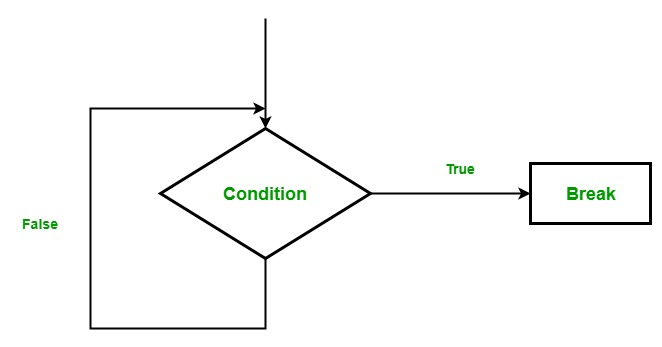# Break statement in Scala

In Scala, we use a break statement to break the execution of the loop in the program. Scala programing language does not contain any concept of break statement(in above 2.8 versions), instead of break statement, it provides a `break `method, which is used to break the execution of a program or a loop. Break method is used by importing `scala.util.control.breaks._ package`.

Flow Chart:Syntax:

```// import package
import scala.util.control._

// create a Breaks object
val loop = new breaks;

// loop inside breakable
loop.breakable{

// Loop starts
for(..)
{
// code
loop.break
}
}
```

or

```import scala.util.control.Breaks._
breakable
{
for(..)
{
code..
break
}
}```

For example:

 `// Scala program to illustrate the  ` `// implementation of break ` ` `  `// Importing break package ` `import` `scala.util.control.Breaks.``_` `object` `MainObject  ` `{ ` `     `  `// Main method ` `def` `main(args``:` `Array[String])  ` `{ ` `     `  `    ``// Here, breakable is used to prevent exception  ` `    ``breakable  ` `    ``{  ` `        ``for` `(a ``<``- ``1` `to ``10``)  ` `        ``{ ` `            ``if` `(a ``==` `6``) ` `         `  `                ``// terminate the loop when  ` `                ``// the value of a is equal to 6 ` `                ``break  ` `            ``else` `             ``println(a); ` `        ``} ` `    ``} ` `} ` `} `

Output:

```1
2
3
4
5```

Break in Nested loop: We can also use break method in nested loop.

For example:

 `// Scala program to illustrate the  ` `// implementation of break in nested loop ` ` `  `// Importing break package ` `import` `scala.util.control.``_` ` `  `object` `Test  ` `{ ` `     `  `// Main method ` `def` `main(args``:` `Array[String])  ` `{ ` `    ``var` `num``1` `=` `0``; ` `    ``var` `num``2` `=` `0``; ` `    ``val` `x ``=` `List(``5``, ``10``, ``15``); ` `    ``val` `y ``=` `List(``20``, ``25``, ``30``); ` ` `  `    ``val` `outloop ``=` `new` `Breaks; ` `    ``val` `inloop ``=` `new` `Breaks; ` `     `  `    ``// Here, breakable is used to  ` `    ``// prevent from exception ` `    ``outloop.breakable  ` `    ``{  ` `        ``for` `(num``1` `<``- x)  ` `        ``{    ` `         `  `            ``// print list x ` `            ``println(``" "` `+ num``1``); ` ` `  `            ``inloop.breakable  ` `            ``{ ` `            ``for` `(num``2` `<``- y)  ` `            ``{ ` `             `  `            ``//print list y ` `            ``println(``" "` `+ num``2``); ` ` `  `            ``if` `(num``2` `==` `25``)  ` `            ``{ ` `                 `  `            ``// inloop is break when  ` `            ``// num2 is equal to 25 ` `            ``inloop.break; ` `            ``} ` `            ``} ` `         `  `        ``// Here, inloop breakable ` `            ``}  ` `        ``} ` `     `  `    ``// Here, outloop breakable ` `    ``}  ` `} ` `} `

Output:

```  5
20
25
10
20
25
15
20
25```

Explanation: In the above example, the initial value of both num1 and num2 is 0. Now first outer for loop start and print 5 from the x list, then the inner for loop start its working and print 20, 25 from the y list, when the controls go to num2 == 25 condition, then the inner loop breaks. Similarly for 10 and 15.

My Personal Notes arrow_drop_upCheck out this Author's contributed articles.

If you like GeeksforGeeks and would like to contribute, you can also write an article using contribute.geeksforgeeks.org or mail your article to contribute@geeksforgeeks.org. See your article appearing on the GeeksforGeeks main page and help other Geeks.

Please Improve this article if you find anything incorrect by clicking on the "Improve Article" button below.

Article Tags :

1

Please write to us at contribute@geeksforgeeks.org to report any issue with the above content.